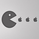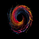Português
TradingView
3832 vizualizações
3832
Wave Channel 3D
Built by Ricardo idea from JR & Aloakdutt from indieTrades Jan. 2010

This indicator is very easy to build. We utilize Moving Averages with a set multiplier and an offset. Specially we try to use Fibonacci sequence series numbers (1, 2, 3, 5, 8, 13, 21, 34, 55, 89, 144...) as time space and multiplier (default 89, 8). Also included is Donchian Channel to locate strong trends and possible future support - resistance.

Examples of support/resistance on chart.

Dominant Price Trends

Future Support Resistance

Comparing Fibonacci Series Time Space - Multiplier

When Comparing make note of confluence support/resistance showing up with Fibonacci Series
Example uses DC

When Comparing make note of confluence support/resistance showing up with Fibonacci Series
Example without DC / Smooth MA
```study(title="3D-Wave Channel", shorttitle="3D-WC", overlay=true)
timespace = input(1)
smooth = input(89)
offsetMultiplier=input(8)
useDonchianAverage = input(false)
src = input(ohlc4)
ma = useDonchianAverage ? avg(highest(smooth),lowest(smooth)) : ema(src, smooth)
plot(ma, color=black, offset=offsetMultiplier*01)
plot(ma[timespace*01], color=silver, offset=offsetMultiplier*02)
plot(ma[timespace*02], color=silver, offset=offsetMultiplier*03)
plot(ma[timespace*03], color=gray, offset=offsetMultiplier*04)
plot(ma[timespace*04], color=gray, offset=offsetMultiplier*05)
plot(ma[timespace*05], color=gray, offset=offsetMultiplier*06)
plot(ma[timespace*06], color=silver, offset=offsetMultiplier*07)
plot(ma[timespace*07], color=silver, offset=offsetMultiplier*08)
plot(ma[timespace*08], color=gray, offset=offsetMultiplier*09)
plot(ma[timespace*09], color=gray, offset=offsetMultiplier*10)
plot(ma[timespace*10], color=black, offset=offsetMultiplier*11)

max_ma()=>max(ma[timespace*10],max(ma[timespace*9],max(ma[timespace*8],max(ma[timespace*7],max(ma[timespace*6],max(ma[timespace*5],max(ma[timespace*4],max(ma[timespace*3],max(ma[timespace*2],max(ma[timespace*1], ma))))))))))
min_ma()=>min(ma[timespace*10],min(ma[timespace*9],min(ma[timespace*8],min(ma[timespace*7],min(ma[timespace*6],min(ma[timespace*5],min(ma[timespace*4],min(ma[timespace*3],min(ma[timespace*2],min(ma[timespace*1], ma))))))))))

top = highest(max_ma(), smooth)
bot = lowest(min_ma(), smooth)

plot(top, color=black, offset=offsetMultiplier)
plot(bot, color=black, offset=offsetMultiplier)```Today maybe it would have been more accurate to use this indicator, my stop loss support was broken too early
Respostasigmadict
Green live VS Grey line
I MISSED THE POP
Respostasigmadict
We can verify with my the S/R I previously draw. It's not far from reality, I recommend to use it as a reference and validate with a line previous S/R more precisely.
Respostasigmadict
An average is not a key level, depending which Time Frame you are using ... you will find the sweet spots in every Timeframes, but if you use it alone on one time frame, it will have some lags
Respostasigmadict
And as a preference, I think it is not mandatory to have all waterfalls, it's visually disturbing the chart
RespostaThank you for sharing this interesting idea. The indicator initially seems to be really great based from the screenshot, but when I tested the signals they are lagging extremely behind the price movement. Even with very fast settings like smooth input(5), offsetMultiplier input(1) I could not find anything I would find useful in actual day-trading.

Don't get me wrong. I think this indicator is great when you analyze a new symbol for the very first time. It is very good to see the overall trend direction of recent weeks; to get an overall feeling where the market was headed recently.
Resposta
PT Português
EN English
EN English (UK)
EN English (IN)
DE Deutsch
FR Français
ES Español
IT Italiano
PL Polski
SV Svenska
TR Türkçe
RU Русский
ID Bahasa Indonesia
MS Bahasa Melayu
TH ภาษาไทย
VI Tiếng Việt
JA 日本語
KO 한국어
ZH 简体中文
ZH 繁體中文
AR العربية
HE עברית